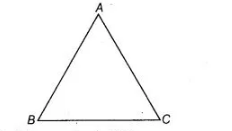# Prove that a triangle must have atleast two acute angles.`
Question:

Prove that a triangle must have atleast two acute angles.

Solution:

Given ΔABC is a triangle.

To prove ΔABC must have two acute angles

Proof Let us consider the following cases

Case I When two angles are 90°.

Suppose two angles are ∠B = 90° and ∠C = 90°We know that, the sum of all three angles is $180^{\circ}$.

$\therefore \quad \angle A+\angle B+\angle C=180^{\circ}$ .....(i)

$\therefore$ $\angle A+90^{\circ}+90^{\circ}=180^{\circ}$

$\Rightarrow$ $\angle A=180^{\circ}-180^{\circ}=0$

So, no triangle is possible.

Case II When two angle are obtuse.

Suppose two angles $\angle B$ and $\angle C$ are more than $90^{\circ}$.

From Eq. (i)

$\angle A=180^{\circ}-(\angle B+\angle C)=180^{\circ}-\left[\right.$ Angle greater than $\left.180^{\circ}\right)$

$\left[\because \angle B+\angle C=\right.$ more than $90^{\circ}+$ more than $90^{\circ}=$ more than $\left.180^{\circ}\right]$

$\angle A=$ negative angle, which is not possible. So, no triangle is possible.

Case III When one angle in $90^{\circ}$ and other is obtuse.

Suppose angle $\angle B=90^{\circ}$ and $\angle C$ is obtuse.

From Eq. (i).

$\angle A+\angle B+\angle C=180^{\circ}$

$\Rightarrow \quad \angle A=180^{\circ}-\left(90^{\circ}+\angle C\right)$

$=90^{\circ}-\angle C$

$=$ Negative angle

$[\because \angle C$ in obtuse $]$

Hence, no triangle is possible.

Case IV When two angles are acute, then sum of two angles is less than $180^{\circ}$, so that the third angle is also acute.